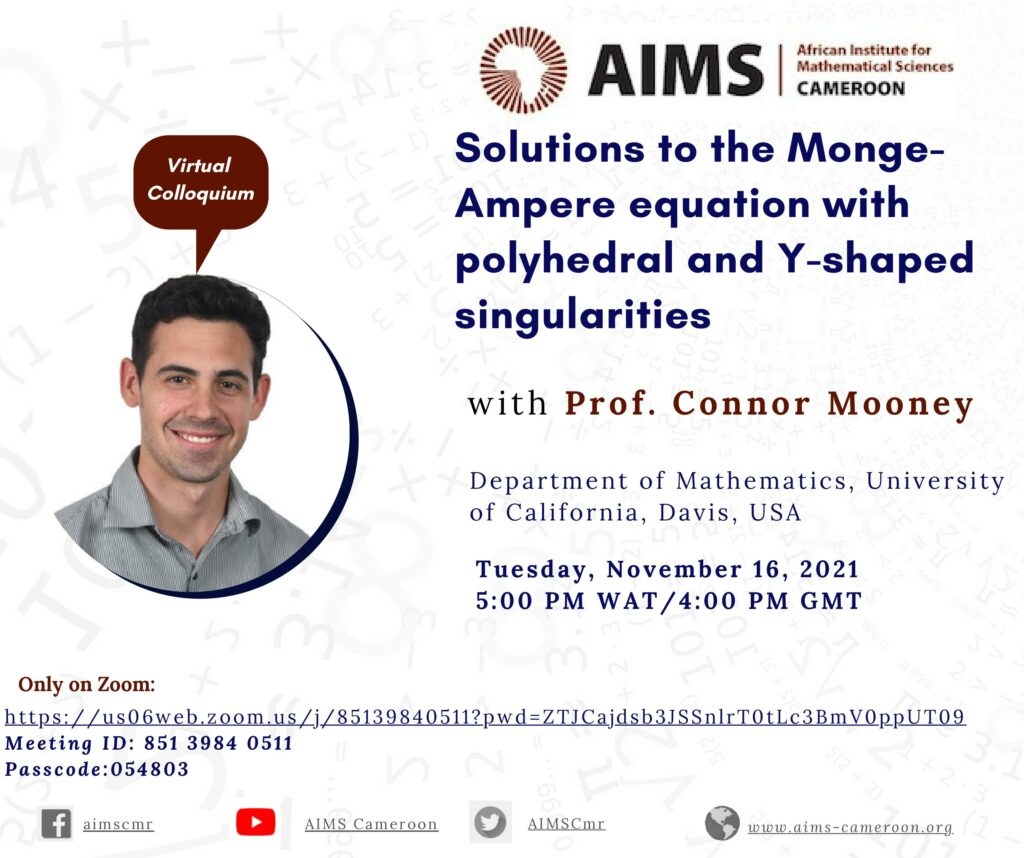November 16, 2021

# Solutions to the Monge-Ampere Equation with Polyhedral and Y-shaped Singularities

Surfaces which The Monge-Ampere equation $\mathrm{det}(D^2u) = 1$ arises in prescribed curvature problems and in optimal transport. An interesting feature of the equation is that it admits singular solutions. We will discuss new examples of convex functions on $\mathbb{R}^n$ that solve the Monge-Ampere equation away from finitely many points but contain polyhedral and $Y$-shaped singular structures.

Along the way, we will discuss geometric and applied motivations for constructing such examples, as well as their connection to a certain obstacle problem.